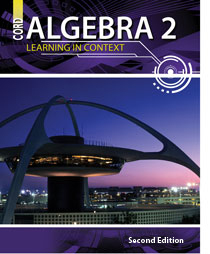# Algebra 2 - 2nd Edition

## Chapter 5: Exponential and Logarithmic Functions

Some links are repeated for use with more than one lesson.

### 5.1 Exponential Functions

http://www.intmath.com/Exponential-logarithmic-functions/Exponential-log-functions-intro.php

### 5.2 Logarithmic Functions

http://www.mathsisfun.com/algebra/logarithms.html
NEW This page presents the concept of logarithms of different bases, with some practice problems.

http://www.purplemath.com/modules/logs.htm
NEW This series of pages present the concept of logarithms,
showing how they relate to exponentials.

### 5.3 Properties of Logarithms

http://www.purplemath.com/modules/logrules.htm
NEW This series of pages presents examples and practice for applying the properties of logarithms.

http://hyperphysics.phy-astr.gsu.edu/Hbase/logm.html

### 5.4 Natural Logarithms

http://betterexplained.com/articles/demystifying-the-natural-logarithm-ln/

http://www.cs.arizona.edu/icon/oddsends/e.htm
This page contains a list of the first 50,000 digits of e.

http://gwydir.demon.co.uk/jo/numbers/interest/e.htm

### 5.5 Solving Exponential and Logarithmic Equations

http://www.sparknotes.com/math/algebra2/logarithmicfunctions/section4.rhtml
This page contains a brief review of solving exponential and logarithmic equations.

http://www.intmath.com/Exponential-logarithmic-functions/6_Logarithm-exponential-eqns.php